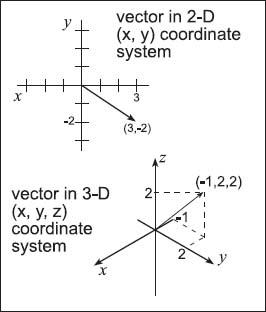# What is a polar coordinate system?

In effect, a polar coordinate system “wraps” a two-dimensional (Euclidean) coordinate system onto the surface of a sphere (for more information about coordinate systems, see “Geometry and Trigonometry”). A polar coordinate system examines a point in space defined in terms of its position and distance on a sphere with a unit radius. The center of the sphere is considered the origin; the first two coordinates are the longitude and latitude on the sphere; the third coordinate defines the distance of the point from the center of the sphere—the values of latitude, longitude, and height. In polar coordinates—it’s easy to see on a globe of our own planet—latitude ranges from +90 to -90, longitude ranges from -180 to +180, and height ranges from zero to infinity. Height can also be negative: The North Pole is at coordinates of (+90, —, +r) (the “—” means there is no longitude), the South Pole is at coordinates of (-90, —, -r), and a point on the equator is at coordinates (0, 0, r).Close

This is a web preview of the "The Handy Math Answer Book" app. Many features only work on your mobile device. If you like what you see, we hope you will consider buying. Get the App